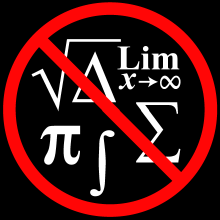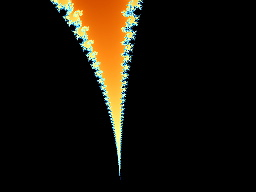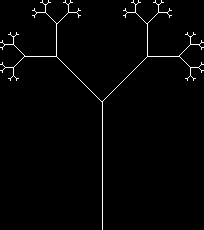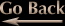Fractals in Plain English by Ivan Freyman

Preface

The internet is awash in articles on how to make fractals. Unfortunately, most of them are loaded with all kinds of Greek characters, squiggly lines, and other mathematical symbols that require a Ph.D in mathematics to understand. Is there a simple way to explain fractals without all this complicated math? Yes, and that has already been done quite well by James Gleick in his book "Chaos: Making a New Science". However, for those who want a much simpler and shorter version, this tutorial should suffice.

What is a Fractal?

A fractal is a mathematically-defined shape which, upon arbitrary magnification, reveals complex structures. For example, a circle is not a fractal because if you magnify it enough, it will look like a straight line. A pentagon is also not a fractal because upon high magnification, it looks like two lines coming together. However, you can magnify a true fractal, such as the Mandelbrot set, forever... as much as your computer's mathematical precision and memory will allow... and you will keep seeing very detailed, complex structures.Magnification: 1Magnification: 5Magnification: 20Magnification: 1Magnification: 5Magnification: 20Magnification: 1Magnification: 5Magnification: 20Magnification: 100Magnification: 500Magnification: 1,000,000

What is the Mandelbrot Set?

The Mandelbrot set was discovered in the late 1970's by Dr. Benoit B. Mandelbrot. It is the set of all complex numbers that fail to escape to infinity after being iterated infinitely many times with the formula Z(n+1)=Z(n)^2+C. Now, what does that cryptic sentence mean in plain English? Well, consider the number zero. If you multiply it by two, you get zero. If you multiply it by two again, you will still get zero. Each multiplication is called an "iteration" by math and programming geeks. No matter how many of these "multiply by two" iterations you perform on zero, you will always have zero. It can be said that zero fails to reach infinity after being iterated infinitely many times with the "multiply by two" formula. However, if you start with any number other than zero, it will grow very quickly until it eventually "escapes" to infinity. For example, if you start with 1, after the first iteration you will have 2, after the second iteration you'll have 4, then 8, 16, and so on. After infinitely many interations, you will have an infinitely large number.

The Mandelbrot formula is only slightly more complicated. You always start with zero, but instead of multiplying something by two, you square it (multiply by itself) and then add a certain number, called a constant. You can choose any constant you want but you must keep it the same while iterating (hence the term 'constant'). For example, let's try the Mandelbrot formula with our constant being -1.5 (negative one point five).

Before iterating, we start with zero. Remember, to get the next value, we square the current value and then add the constant.

Iteration One: Square zero. This still gives us zero, but now we add our constant -1.5. We get a total of -1.5.

Iteration Two: Square -1.5. This gives us 2.25 and again we add our constant -1.5. This gives a total of 0.75.

Iteration Three: Square 0.75. This gives us .5625, and again we add the constant -1.5 which gives a new total of -0.9375.

Iteration Four: Square -0.9375... etc.

We repeat this process of squaring and adding -1.5 many times. What do you think will happen? Well, the number doesn't approach zero, and it doesn't escape to infinity. It keeps changing chaotically with every iteration and there is no discernable pattern to the changes. After a hundred iterations, the number is about -0.248. After a thousand iterations, it's about 0.651 and after a million it's -0.2255.

Depending on what constant you use, Mandelbrot's formula will either escape to infinity or chaotically change forever (as we saw with -1.5). There is a third possibility: It can slowly approach some fixed number. For example if your constant is 0.25 you will slowly approach 0.5. The more you iterate with a constant of 0.25, the closer you get to 0.5. No chaotic bouncing, no infinity... just getting closer and closer to a number.

So how does all this apply to the pretty pictures of the Mandelbrot set? Well, here it gets a bit more complicated. The Mandelbrot set calculations take place in the complex plane. If you don't know what that is, please Google it and find out because otherwise you won't understand what follows. When Mandelbrot pictures are created, instead of using a simple constant like we used before, we use a complex constant, for example: 1.5+2i or 0.5-0.25i.

To make a Mandelbrot set, consider the region of the complex plane that extends from -2 to +2 on both axes. Take a point from this region and use it as a constant in the Mandelbrot formula described above. If it escapes to infinity, draw a white pixel in the location where you got the point. If it does not escape to infinity, draw a black pixel there. Repeat this process for every point in the region. When you're finished, the black squares will be the Mandelbrot set.

What are Newton's Complex Boundaries?

Sir Isaac Newton discovered a way to find the roots of any complex polynomial. Unfortunately, he did not live to see the incredible fractals that his equations can produce. Newton's fractal uses a completely different technique from Mandelbrot. It involves some calculus and at a minimum, a third-degree complex polynomial. Take an equation, such as Z^3=1 (Z cubed equals 1). With real numbers, there is only one solution for Z, and that is Z=1. With complex numbers, there are three solutions. I won't get into the math behind Newton's formula because it is considerably more complex than Mandelbrot and is beyond the scope of this tutorial. However, here is how it works: If you want to find the three values of Z that satisfy the equation Z^3=1, you start with some point in the complex plane and iterate using Newton's root-finding algorithm. Eventually, the algorithm will converge on one of the roots. You color the point of your starting location in proportion to the number of iterations it took to find the root. You do this for every point on the complex between -2 and +2 on both axes. The strange thing is that for some polynomials, the fractals contain small copies of the Mandelbrot set! This is incredible because the Mandelbrot formula is only a second-degree equation (Z^2+C) and uses a completely different algorithm from Newton. Yet, they both produce the same fractal shapes.

What are Iterated Function Systems?

Iterated Function Systems (IFS) are much simpler to make than Mandelbrot or Newton fractals, but they're also completely self-similar and therefore not very interesting when magnified. It's better to look at them under low magnification because higher magnifications do not reveal any new details or structures. Here is how you can make a simple IFS tree using a pencil and a sheet of paper:

1. Start from the bottom of the page.
2. Move forward 1 inch.
3. Choose a random direction: left or right. Turn 45 degrees in that direction
4. Move in this new direction half the distance you previously moved.
5. Repeat steps 3 and 4 a few times. You just drew one branch of the tree.
6. Goto step 1. You will now be adding more branches. Keep doing this until you get tired.A simple IFS Tree